Most Affordable JEE | NEET | 8,9,10 Preparation by Kota's Top IITian Doctor Faculties

# s block Elements Class 11 Important Questions with AnswersGet s-block elements important questions with detailed answers for class 11 exams preparation. View the Important Question bank for Class 11 and class 12 Chemistry complete syllabus. These important questions and answers will play significant role in clearing concepts of Chemistry class 11. This question bank is designed keeping NCERT in mind and the questions are updated with respect to upcoming Board exams. You will get here all the important questions for class 11 chemistry chapters. Learn the concepts of s block elements and other topics of chemistry class 11 and 12 syllabus with these important questions and answers along with the notes developed by experienced faculty. Click Here for Detailed Chapter-wise Notes of Chemistry for Class 11th, JEE & NEET.  You can access free study material for all three subject’s Physics, Chemistry and Mathematics. Click Here for Detailed Notes of any chapter.

eSaral provides you complete edge to prepare for Board and Competitive Exams like JEE, NEET, BITSAT, etc. We have transformed classroom in such a way that a student can study anytime anywhere. With the help of AI we have made the learning Personalized, adaptive and accessible for each and every one. Visit eSaral Website to download or view free study material for JEE & NEET. Also get to know about the strategies to Crack Exam in limited time period. Very Short Answer (1 Mark)

Q.. Why first group elements are called alkali metals ?
Ans. Group I elements are highly reactive and with water (moisture in the atmosphere) form strong alkalies, so they are called alkali metals.
Q.. Write the chemical name and formula of washing soda.
Ans. Washing soda is sodium carbonate $\left(N a_{2} C O_{3}\right)$.
Q.. Why do alkali metals not occur in free state ?
Ans. They are highly reactive, therefore, they occur in combined state and do not occur in free state.
Q.. Write the important minerals of lithium.
Ans. The important minerals of lithium are:
(i) Lipidolite $(L i . N a . K)_{2} A l_{2}\left(S i O_{3}\right)_{3} \cdot F(O H)$ (ii) Spodumene $L i A I S i_{2} O_{3}$ (iii) Amblygonite $\operatorname{LiAl}\left(P O_{4}\right) F$
Q.. How sodium hydroxide is prepared at large scale ?
Ans. At large scale, sodium hydroxide is prepared by castner kellner cell.

Q.. Why $\mathrm{KHCO}_{3}$ is not prepared by Solvay process ?
Ans.Because solubility of $\mathrm{KHCO}_{3}$ is fairly large as compared to $\mathrm{NaHCO}_{3}$.
Q.. What is chemical composition of the Plaster of Paris ?
Ans. Plaster of paris is calcium sulphate hemihydrate : $\mathrm{CaSO}_{4} \cdot \frac{1}{2} \mathrm{H}_{2} \mathrm{O}$ or $\left(\mathrm{CaSO}_{4}\right)_{2} \cdot \mathrm{H}_{2} \mathrm{O}$
Q.. Why do alkali metals have low density ?
Ans. Due to weak metallic bonds and large atomic size, their density is low.
Q.. Why is first ionization energy of alkali metals lower than those of alkaline earth metals ?
Ans. Alkali metals have bigger atomic size, therefore they have lower first I.E. than group 2 elements.
Q.. First group elements are strong reducing agents, why ?
Ans. Because they have a strong tendency to lose outer most electron.
Q.. Explain why ? LiI is more soluble than KI in ethanol. [NCERT]
Ans. $K I$ In the chemical bond is ionic in character. On the other hand due to small size of lithium ion and its high polarising power the bond in is predominently covalent in character. Hence LiI is more soluble than in ethanol.
Q.. LiH is more stable than $\mathrm{NaH}$ Explain.
Ans. Both $L i^{+}$ and $H^{-}$ have small size and their combination has high lattice energy. Therefore LiH is stable as compared with $\mathrm{NaH}$
Q.. Why is $B e C l_{2}$ soluble in organic solvents ?
Ans. $B e C l_{2}$ is covalent, therefore soluble in organic salvents.
Q.. Name the metal which floats on water without any apparent reaction with water. [NCERT]
Ans. Lithium floats on water without any apparent reaction with it.
Q.. Name an element which is invariably bivalent acid and whose oxide is soluble in excess of NaOH and its dipositive ion has a noble gas core.
Ans. The element is beryllium, its oxide $B e O$ is soluble in excess of $\mathrm{NaOH}$ $B e O+2 N a O H \longrightarrow N a_{2} B e O_{2}+H_{2} O$ Its dipositive ion has electronic configuration $\left(B e^{2+}=1 s^{2}\right)$
Q.. State reason for the high solubility of $B e C l_{2}$ in organic solvents.
Ans. Because $B e C l_{2}$ is covalent compound.
Q.. What is the cause of diagonal relationship ? [NCERT]
Ans. The charge over radius ratio, i.e., polarizing power is similar, that is the cause of diagonal relationship.
Q.. Name the alkali metals which forms superoxide when heated in excess of air.
Ans. Potassium, Rubidium and caesium form superoxide when heated in excess of air.
Q.. Which out of K, Mg,Ca & Al form amphoteric oxide ?
Ans. form amphoteric oxide, i.e., acids as well as basic in nature.
Q.. Explain the following : Sodium wire is used to dry benzene but cannot be used to dry ethanol.
Ans. Sodium metal removes moisture from benzene by reacting with water. However, ethanol cannot be dried by using sodium because it reacts with sodium. $2 \mathrm{Na}+\mathrm{C}_{2} \mathrm{H}_{5} \mathrm{OH} \longrightarrow 2 \mathrm{C}_{2} \mathrm{H}_{5} \mathrm{ONa}+\mathrm{H}_{2}$
Q.. Why is $\mathrm{CaCl}_{2}$ added to $N a C l$ in extraction of $N a$ by Down cell ?
Ans. $\mathrm{CaCl}_{2}$ reduces melting point of $N a C l$ and increases electrical conductivity.
Q.. Carbon dioxide is passed through a suspension of limestone in water. Write balanced chemical equation for the above reaction.
Ans. $\mathrm{CaCO}_{3}+\mathrm{H}_{2} \mathrm{O}+\mathrm{CO}_{2} \longrightarrow \mathrm{Ca}\left(\mathrm{HCO}_{3}\right)_{2}$
Q.. What do we get when crystals of washing soda exposed to air ?
Ans. We get amorphous sodium carbonate becouse it loses water molecules.
Q.. $M g_{3} N_{2}$ when react with water gives off $\mathrm{NH}_{3}$ but $H C l$ is not obtained from $M g C l_{2}$on reaction with water at room temperature.
Ans. $M g_{3} N_{2}$ is a salt of a strong base, $M g(O H)_{2}$ and a weak acid $\left(N H_{3}\right)$ and hence gets hydrolysed to give $N H_{3} .$ In contrast, $M g C l_{2}$ is a salt of a strong base, and a strong acid, and hence does not undergo hydrolysis to give
Q.. Why caesium can be used in photoelectric cell while lithium cannot be ?
Ans. Caesium has the lowest while lithium has the highest ionization enthalpy among all the alkali metals. Hence, caesium can lose electron very easily while lithium cannot.
Q.. Which nitrates are used in pyrotechnics ?
Ans. Strontium and Barium nitrates are used in pyrotechnics for giving red and green flames.
Q..What are s-block elements ? Write their electronic configuration.
Ans. The elements in which the last electron enters the -orbital of their outermost energy level are called -block elements. It consists of Group 1 and Group 2 elements. Their electronic configuration is
Q.. Name the metals which are found in each of the following minerals : (i) Chile Salt Petre (ii) Marble (iii) Epsomite (iv) Bauxite.
Ans. (i) $\quad N a$(ii) $\mathrm{Ca}$ (iii) $M g \quad$ (iv) $\quad A l$
Q.. What is composition of Portland cement ? What is average composition of good quality cement ? [NCERT]
Ans. $\mathrm{CaO}=50$ to $60 \% \quad \mathrm{SiO}_{2}=20$ to $25 \%, \quad \mathrm{Al}_{2} \mathrm{O}_{3}=5$ to $10 \%$ $M g O=2$ to $3 \%, \quad F e_{2} O_{3}=1$ to $2 \%, \quad S O_{2}=1 \quad$ to $2 \% \quad$ is The ratio of $S i O_{2}$ (silica) to alumina $\left(A l_{2} O_{3}\right)$ should be between 2.5 and 4.0 and the ratio of lime $(\mathrm{CaO})$ to total oxides of silicon $\mathrm{SiO}_{2}, \mathrm{Al}_{2} \mathrm{O}_{3}$ and $\mathrm{Fe}_{2} \mathrm{O}_{3}$ should be as close to 2 as possible.
Q.. Write chemical reactions involved in Down process for obtaining Mg from sea water.
Ans.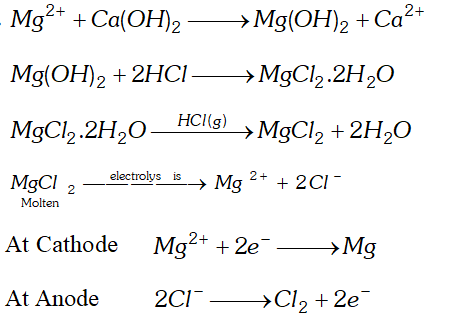Q.. What is the mixture of $\mathrm{CaCN}_{2}$ and carbon called ? How is it prepared ? Give its use.
Ans.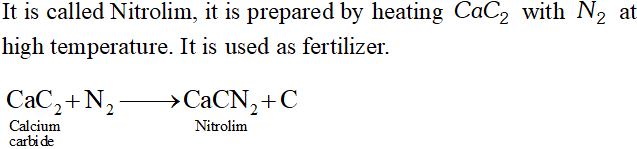Q.. State the difficulties in extraction of alkaline earth metals from the natural deposits.
Ans. Like alkali metals, alkaline earth metals are also highly electropositive and strong reducing agents. Same difficulties, we face in the extraction of these metals. Therefore, these metals are extracted by electrolysis of their fused metal halides.
Q.. Starting with sodium chloride how would you proceed to prepare (state the steps only) : (i) sodium metal (ii) sodium hydroxide (iii) sodium peroxide (iv) sodium carbonate [NCERT]
Ans.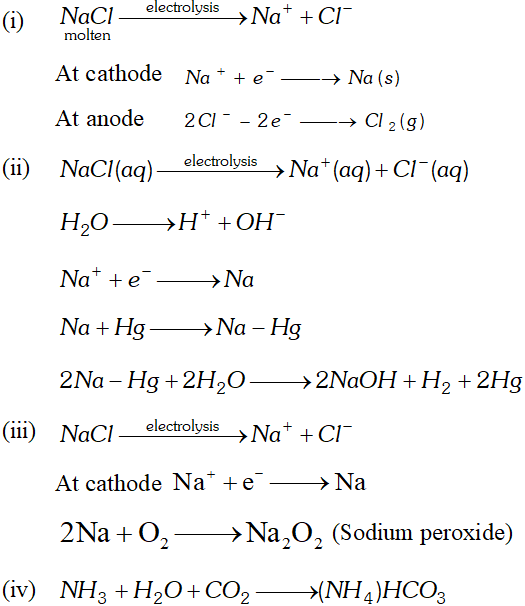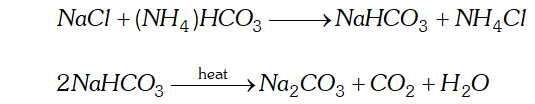Q..Write three general characteristics of the elements of s-block of the periodic table which distinguish them from the elements of the other blocks.   [NCERT]
Ans. (i) They do not show variable oxidation states. (ii) They are soft metals having low melting and boiling point. (iii) They are highly electropositive and most reactive metals.
Q.. Why is $L i F$ almost insoluble in water whereas $L i C l$ is soluble not only in water but also in acetone ?   [NCERT]
Ans. $L i F$ is an ionic compound containing small ions and hence has very high lattice enthalpy. Enthalpy of hydration in this case is not sufficient to compensate for high lattice enthalpy. Hence, is insoluble in water. has partial covalent character due to polarization of chloride ion by ion. Thus, has partial covalent character and partial ionic character and hence is soluble in water as well as less polar solvents such as acetone.
Q.. When an alkali metal dissolves in liquid ammonia the solution acquires different colours. Explain the reasons for this type of colour change. [NCERT]
Ans. When an alkali metal is dissolved in liquid ammonia it produces a blue coloured conducting solution due to formation of ammoniated cation and ammoniated electron as given below :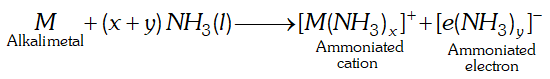When the concentration is above the colour of solution is copper-bronze. This colour change is because the concentrated solution contains clusters of metal ions and hence possess metallic lustre.
Q.. Arrange the following in the decreasing order of the property mentioned :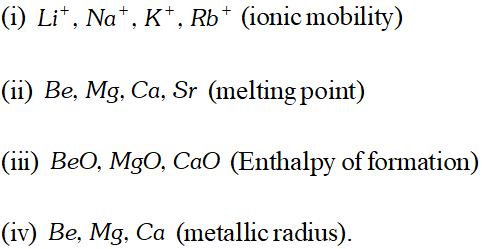Ans.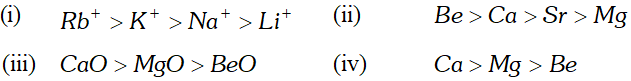Q.. Alkali metals have low ionisation enthalpies.Why is it so?
Ans. Alkali metals have larger atomic size, therefore they have lower ionisation enthalpies. There is less force of attraction between valence electron and nucleus, therefore less energy is required to remove electron.
Q.. Which out of $L i, N a, K, B e, M g, C a$ has lowest ionisation enthalpy and why ?
Ans. $K^{\prime}$ has lowest ionisation energy due to larger atomic size among these elements. The force of attraction between valence electron and nucleus is less, therefore it can loose electron easily.
Q.. What is responsible for the blue colour of the solution of alkali metal in liquid ammonia ? Give chemical equation also.
Ans. The solvated electron, $e\left(N H_{3}\right)_{x}$ or ammoniated electron is responsible for blue colour of alkali metal solution in It absorbs light from visible regions and radiates complimentary colour: $2 N a(s)+2 N H_{3}(l) \longrightarrow 2 N a N H_{2}(s)+H_{2}(g)+e\left(N H_{3}\right)_{x}$
Q.. The alkali metals follow the noble gases in their atomic structure. What properties of these metals can be predicted from the information ?
Ans. (i) They form unipositive ions. (ii) Their second ionisation energy is very high. (iii) They have weak metallic bonds due to larger size and only one valence electron.
Q.. Comment on each of the following observations : (i) The mobilities of the alkali metal ions in aqueous solution are $L i^{+} Ans. (i)$L i^{+}$ions are smallest in size therefore most hydrated, that is why they have lowest mobility in aqueous solution.$C s^{+}$ions are largest in size, least hydrated, therefore have highest mobility. Size of hydrated cation decreases, therefore, mobility of ions increases down the group. (ii)$L i$is smallest in size and best reducing agent, therefore, it forms nitride with$N_{2}6 L i+N_{2} \longrightarrow 2 L i_{3} N$(Lithium nitride) (iii) It is due to less difference in their standard reduction potentials which is resultant of sublimation energy, ionisation energy and hydration energy. (iv) is least ionic as compared to other fluorides of alkali metals. It has high lattice energy, therefore, it is least soluble. Q.. Why do alkali metals impart characteristic colours to the flame of a bunsen burner ? What is the colour imparted to the flame by each of the following metals ? Lithium, Sodium and Potassium. Ans. When the alkali metal or any of its compounds is introduced into a flame, the electrons absorb energy from flame and get excited to higher energy levels. When these electrons come to ground state, the absorbed energy is given out in the form of radiations in the visible region. Lithium imparts caramine red, sodium gives golden yellow and potassium gives pink violet colour to the flame. Q.. Which one of the alkaline earth metal carbonate is thermally most and least stable and why ? Ans.$B a C O_{3}$is thermally most stable due to greater ionic character and high lattice energy where as$B a C O_{3}$is thermally least stable because it is covalent and has less lattice energy. Q.. Commercial aluminium always contains some magnesium. Name two such alloys of aluminium. What properties are imparted by the addition of magnesium in these alloys ? [NCERT] Ans. Duralium and Magnaliam are alloys of$\mathrm{Al}, \mathrm{Mg}$is lighter in density than$A l$therefore, it makes the alloys lighter. These alloys are used in automobile engines and aeroplanes. Q.. Arrange the (i) hydroxides and (ii) Sulphates of alkaline earth metals in order of decreasing solubilities., giving a suitable reason for each. Ans.$-B e(O H)_{2}M g S O_{4}>C a S O_{4}>S r S O_{4}>B a S O_{4}$Solubility of sulphate goes on decreasing down the group because lattice energy dominates over hydration energy. Q.. State the properties of beryllium different than other elements of the group. Ans. Properties of beryllium different than other elements of the group (i) Beryllium is harder than other elements. (ii) Melting and boiling points are higher than that of other elements. (iii) It does not react with water but other elements do. (iv) It does not react with acids to form hydrogen. (v) It forms covalent compounds but others form ionic compounds. (vi) Beryllium oxide is amphoteric but other oxides are basic. Q.. Mention the general trends in Group 1 and in Group 2 with increasing atomic number with respect to (i) density (ii) melting point (iii) atomic size (iv) ionization enthalpy. [NCERT] Ans.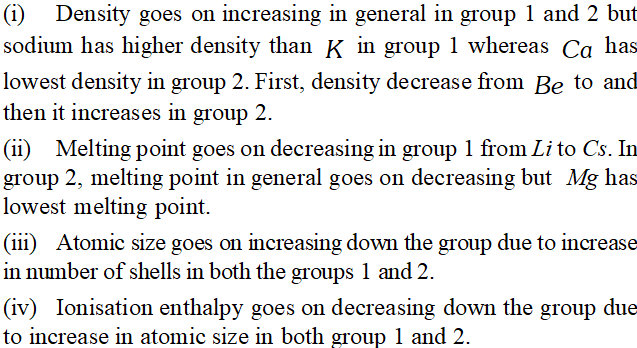Q.. How do the following properties change on moving from Group 1 to Group 2 in the periodic table ? (i) Atomic size (ii) Ionization enthalpy (iii) Density (iv) Melting points. Ans. (i) Atomic size decrease from group 1 to group 2 due to increase in effective nuclear charge. (ii) First ionisation enthalpy increases from group 1 to group 2 due to decrease in atomic size. (iii) Density increases from group 1 to 2. (iv) Melting points increase from group 1 to 2. Q.. Compare and contrast the chemistry of Group 1 metals with that of Group 2 metals with respect to (i) nature of oxides (ii) solubility and thermal stability of carbonates (iii) polarizing power of cations (iv) reactivity and reducing power. Ans. (i) Group 1 metals form oxides of strong basic nature (except$L i_{2} O$. Group 2 metal form oxide of less basic nature. (ii) Carbonates : Carbonates of Group 1st are soluble and stable, solubility in case of Group 2nd decreases down the group. (iii) Polarizing power of cations increases. (iv) Reactivity and reducing power decreases. Q.. Explain what happens when ? (i) Sodium hydrogen carbonate is heated (ii) Sodium amalgam reacts with water (iii) Fused sodium metal reacts with ammonia. Ans. (i) On heating sodium bicarbonate it forms sodium carbonate $2 \mathrm{NaHCO}_{3} \stackrel{\Delta}{\longrightarrow} \mathrm{Na}_{2} \mathrm{CO}_{3}+\mathrm{H}_{2} \mathrm{O}+\mathrm{CO}_{2}$ (ii) When sodium amalgam,$N a / H g,$is formed the vigourosity of reaction of sodium with water decreases. $2 \mathrm{Na} / \mathrm{Hg}+2 \mathrm{H}_{2} \mathrm{O} \longrightarrow \mathrm{NaOH}+\mathrm{H}_{2}$ (iii) Sodium reacts with ammonia to form amide. $2 \mathrm{Na}+2 \mathrm{NH}_{3} \stackrel{\Delta}{\longrightarrow} 2 \mathrm{NaNH}_{2}+\mathrm{H}_{2}$ Q.. State as to why ? (i) An aqueous solution of sodium carbonate give alkaline tests.$\mathrm{Na}_{2} \mathrm{CO}_{3}+\mathrm{H}_{2} \mathrm{O} \longrightarrow \mathrm{Na}^{+}+\mathrm{OH}^{-}+\mathrm{HCO}_{3}^{-}$(ii) Sodium is prepared by electrolytic method and not by chemical method. Ans. (i) An aqueous solution of sodium carbonate has a large concentration of hydroxyl ions making it alkaline in nature. (ii) Sodium is a very strong reducing agent therefore it cannot be extracted by the reduction of its ore (chemical method). Thus the best way to prepare sodium is by carrying electrolysis of its molten salts containing impurities of calcium chloride. Q.. Explain why : (i) Lithium on being heated in air mainly forms the monoxide and not peroxide. (ii)$K, R b$and$C s$on being heated in the presence of excess supply of air form superoxides in preference to oxides and peroxides. (iii) An aqueous solution of sodium carbonate is alkaline in nature. Ans. (i)$L i^{+}$ions is smaller in size. It is stabilized more by smaller anion, oxide ion$\left(O^{2-}\right)$as compared to larger anion, peroxide ion$\left(O_{2}^{2-}\right)$. (ii)$\quad K^{+}, R b^{+}, C s^{+},$are large cations. A large cation is more stabilized by large anions. since superoxide ion,$O_{2}^{-}$is quite large,$K, R b$and$C s$form superoxides in preference to oxides and peroxides. (iii) In aqueous solution sodium carbonate undergoes hydrolysis forming sodium hydroxide.$\mathrm{Na}_{2} \mathrm{CO}_{3}+\mathrm{H}_{2} \mathrm{O} \longrightarrow \mathrm{NaHCO}_{3}+\mathrm{NaOH}$Q.. Write balanced equations or reactions between :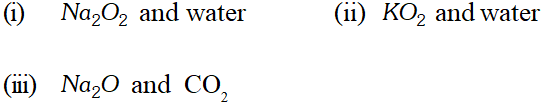Ans.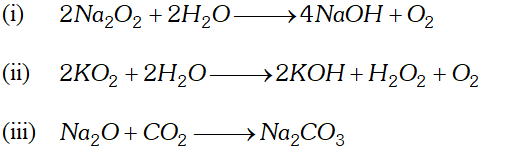Q.. Name an alkali metal carbonate which is thermally unstable and why ? Give its decomposition reaction. Ans.$L i_{2} C O_{3}$is thermally unstable because it is covalent. It decomposes to form$L i_{2} O$and$C O_{2}L i_{2} C O_{3} \stackrel{\Delta}{\longrightarrow} L i_{2} O+C O_{2}$Q.. Why are ionic hydrides of only alkali metals and alkaline earth metals are known ? Give two examples. Ans. Alkali metals and alkaline earth metals are most electropositive due to low ionisation energy or enthalpy therefore, they can form ionic hydrides e.g.,$\mathrm{NaH}, \mathrm{KH}$and$\mathrm{CaH}_{2}$Q.. Why does the following reaction :proceed better with$K F$than with$N a F ?$Ans. It is because$K F$is more ionic than$N a F$Q.. The enthalpy of formation of hypothetical$\operatorname{CaCl}(s)$theoretically found to be equal to$188 \mathrm{kJ} \mathrm{mol}^{-1}$and the$\Delta H_{f}^{\circ}$for$C a C l_{2}(s)$is$-795 k J m o l^{-1} .$Calculate the$\Delta H^{\circ}$for the disproportionation reaction. Ans.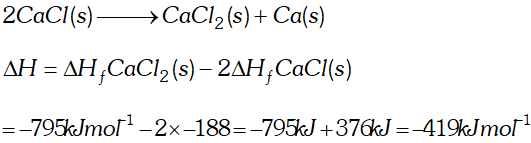Q.. Why is it that the s-block elements never occur in free state in nature ? What are their usual modes of occurrence and how are they generally prepared ? [NCERT] Ans. s-block elements are highly reactive, therefore they never occur in free state rather occur in combined state in the form of halides, carbonates, sulphates. They are generally prepared by electrolysis of their molten salts . Q.. Name the chief form of occurrence of magnesium in nature. How is magnesium extracted from one of it ores ? [NCERT] Ans. mg occurs in the form of$M g C l_{2}$in sea water from which it can be extracted. Sea water containing$\mathrm{MgCl}_{2}$is concentrated under the sun and is treated with$\mathrm{Ca}(\mathrm{OH})_{2} \cdot \mathrm{Mg}(\mathrm{OH})_{2}$is thus precipitated, filtered and heated to give the oxide. The oxide is treated with$\mathrm{C}$and$\mathrm{Cl}_{2}$to get$\mathrm{MgCl}_{2}$.$\mathrm{MgO}+\mathrm{C}+\mathrm{Cl}_{2} \stackrel{\text { heat }}{\longrightarrow} \mathrm{MgCl}_{2}+\mathrm{CO}\mathrm{MgCl}_{2}$is fused with$\mathrm{NaCl}$and$\mathrm{CaCl}_{2}$at$970-1023 \mathrm{K}$and molten mixture is electrolysed. Magnesium is liberated at cathode and Chlorine is evolved at the anode. At Cathode :$\mathrm{Mg}^{2+}+2 \mathrm{e}^{-} \longrightarrow \mathrm{Mg}$At Anode :$2 \mathrm{Cl}^{-} \longrightarrow \mathrm{Cl}_{2}+2 \mathrm{e}^{-}$A steam of coal gas is blown through the cell to prevent oxidation of Mg metal. Q.. How is pure magnesium prepared from sea water ? What happens when mg is burned in air ? Write chemical equations of reactions involved. Ans. Sea water contains$M g C l_{2}$which is concentrated under the sun and is treated with calcium hydroxide,$\mathrm{Ca}(\mathrm{OH})_{2}$, magnesium hydroxide is thus precipitated, filtered and heated to give oxide. The oxide is treated with carbon and$C l_{2}$to get$M g C l_{2}$.$M g O+C+C l_{2} \longrightarrow M g C l_{2}+C O$is mixed with sodium chloride so as to reduce its melting point and increase its electrical conductivity. Molten mixture is electrolysed using steel cathode and carbon anode. A steam of coal gas is blown through the cell to prevent oxidation. of metal. At cathode Mg't$+2 \mathrm{e}^{-} \longrightarrow \mathrm{Mg}$At anode$\quad 2 \mathrm{Cl}^{-}-2 \mathrm{e}^{-} \longrightarrow \mathrm{Cl}_{2}$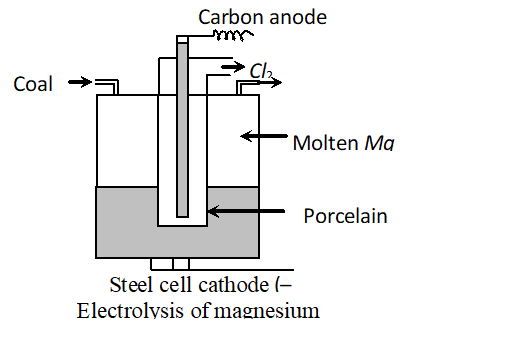mg obtained in liquid state is further distilled to give pure mg When burns in air, it forms magnesium oxide and magnesium nitride.$3 M g+N_{2} \longrightarrow M g_{3} N_{2} ; 2 \mathrm{Mg}+\mathrm{O}_{2} \longrightarrow 2 \mathrm{MgO}$Q.. (i) Draw a neat and labelled diagram of Castner-Kellner cell for the manufacture of caustic soda. (ii) Give chemical equations of the reaction of caustic soda with (a) ammonium chloride, and (b) carbon dioxide. Ans.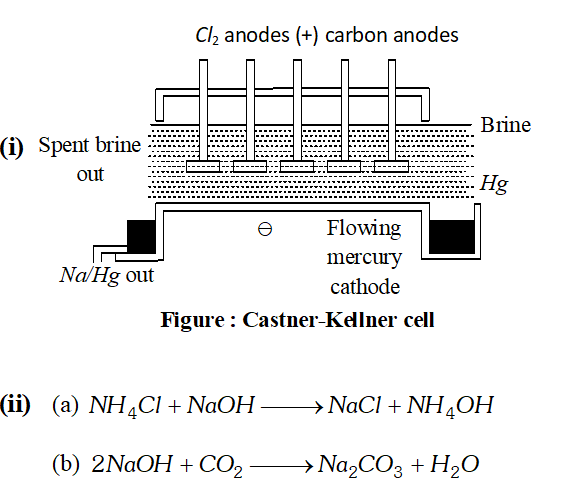Q.. List three properties of lithium in which it differs from the rest of the alkali metals. [NCERT] Ans. (i) Lithium reacts with$N_{2}$to form Lithium nitride whereas other alkali metals do not react with . (ii) Lithium forms monoxide whereas other elements form peroxide and super oxide. (iii) Lithium predominently forms covalent compounds whereas others form ionic compounds. Q.. What happens when : (i) sodium metal is dropped in water. (ii) sodium metal is heated in free supply of air. (iii) sodium peroxide dissolves in water. Ans. (i)$2 N a+2 H_{2} O \longrightarrow 2 N a O H+H_{2}$; sodium hydroxide is formed with evolution of$H_{2}(g)$The hydrogen gas catches fire due to highly exothermic process. (ii)$\quad 2 \mathrm{Na}+\mathrm{O}_{2} \longrightarrow \mathrm{Na}_{2} \mathrm{O}_{2}$sodium peroxide is formed. (iii) Sodium hydroxide and hydrogen peroxide are formed.$\mathrm{Na}_{2} \mathrm{O}_{2}+2 \mathrm{H}_{2} \mathrm{O} \longrightarrow 2 \mathrm{NaOH}+\mathrm{H}_{2} \mathrm{O}_{2}$Q.. The hydroxides and carbonates of sodium and potassium are easily soluble in water while the corresponding salts of magnesium and calcium are sparingly soluble in water. Explain. Ans. The lattice enthalpies of hydroxides and carbonates of magnesium and calcium are very high due to the presence of divalent cations. The enthalpy of hydration cannot compensate for the energy required to break the lattice in these compounds. Hence, they are sparingly soluble in water. On the otherhand, the lattice enthalpies of hydroxides and carbonates of sodium and potassium are low due to the presence of monovalent cations. The enthalpy of hydration in this case is sufficient to break the lattice in these compounds. Hence, hydroxides and carbonates of sodium and potassium are easily soluble in water. Q.. What happens when : (i) magnesium is burnt in air (ii) quicklime is heated with silica (iii) chlorine reacts with slaked lime (iv) calcium nitrate is heated [NCERT] Ans.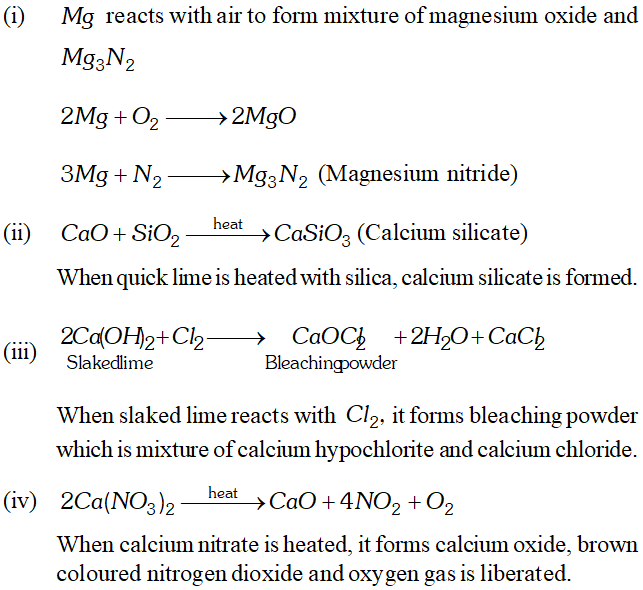Q.. Like lithium in Group 1, beryllium shows anomalous behaviour in Group 2. Write three such properties of beryllium which make it anomalous in the group. Ans. (i) Be forms amphoteric oxide whereas others form basic acids. (ii)$B e C l_{2}$is covalent, others form ionic halides. (iii) does not react even with hot water where as others react easily with water. has smallest atomic size, highest ionisation energy, high polarising power which makes it anomalous in this group. Q.. Beryllium exhibits some similarities with aluminium. Point out three such properties. [NCERT] Ans. (i)$\quad B e O$and$A l_{2} O_{3}$are amphoteric. (ii)$\quad$Be and$A l$react with$\mathrm{NaOH}$to form$\left[\mathrm{Be}(\mathrm{OH})_{2}\right]^{-2}$and$\left[A l(O H)_{6}\right]^{3-}$(iii)$\quad B e_{2} C$and$A l_{4} C_{3}$react with$H_{2} O$to form methane. Q.. Compare the solubility and thermal stability of the following compounds of the alkali metals with those of the alkaline earth metals : (i) nitrates (ii) carbonates (iii) sulphates. Ans. (i) Nitrates of alkali metals are more stable than that of alkaline earth metals. All nitrates are soluble in water. (ii) Carbonates of alkali metals are more stable and more soluble in water than that of alkaline earth metals. (iii) Sulphates of alkali metals are more stable and more soluble in water than that of alkaline earth metal. Q.. Discuss the anomalous behaviour of Lithium. Give its diagonal relationship with Magnesium. Ans.$L i$shows anomalous behaviour due to smallest size, highest ionisation energy and highest polarising power Resemblance with : (i) Both react with$N_{2}$to form nitrides. (ii) Both form predominantly covalent compounds (iii) Both form monoxide (iv) Carbonates of both are thermally unstable. Q.. State, why : (i) A solution of$\mathrm{Na}_{2} \mathrm{CO}_{3}$is alkaline. (ii) Alkali metals are prepared by electrolysis of their fused chlorides. (iii) Sodium is found more useful than potassium. Ans. (i)$\mathrm{Na}_{2} \mathrm{CO}_{3}$is alkaline due to its hydrolysis, it forms$O H^{-}$more than$H^{+}$because$H_{2} C O_{3}$is weak acid, therefore, is alkaline. (ii) Alkali metals are strong reducing agents and highly reactive with water, therefore, they are prepared by electrolysis of their fused Chlorides. (iii) Sodium is more useful than potassium because sodium is less reactive and found in more abundance than K Q.. Contrast the action of heat on the following : (i)$\quad \mathrm{Na}_{2} \mathrm{CO}_{3}$and$\mathrm{CaCO}_{3}$(ii)$\quad M g C l_{2} \cdot 6 H_{2} O$and$C a C l_{2} \cdot 6 H_{2} O$(iii)$\quad \mathrm{Ca}\left(\mathrm{NO}_{3}\right)_{2}$and$\mathrm{NaNO}_{3}$Ans. (i) Sodium carbonate does not decompose whereas calcium carbonate decomposes on heating.$\quad \mathrm{CaCO}_{3} \stackrel{\Delta}{\longrightarrow} \mathrm{CaO}+\mathrm{CO}_{2}$(ii)$\mathrm{MgCl}_{2} \cdot 6 \mathrm{H}_{2} \mathrm{O} \stackrel{\Delta}{\longrightarrow} \mathrm{MgO}+2 \mathrm{HCl}+5 \mathrm{H}_{2} \mathrm{O}\mathrm{CaCl}_{2} \cdot 6 \mathrm{H}_{2} \mathrm{O} \longrightarrow \mathrm{CaCl}_{2}+6 \mathrm{H}_{2} \mathrm{O}$(iii)$2 \mathrm{Ca}\left(\mathrm{NO}_{3}\right)_{2} \stackrel{\Delta}{\longrightarrow} 2 \mathrm{CaO}+4 \mathrm{NO}_{2}+\mathrm{O}_{2}2 \mathrm{NaNO}_{3} \stackrel{\Delta}{\longrightarrow} 2 \mathrm{NaNO}_{2}+\mathrm{O}_{2} |$Q.. What happens when : (i) Carbon dioxide gas is passed through an aqueous solution of sodium carbonate. (ii) Potassium carbonate is heated with milk of lime. (iii) Lithium nitrate is heated. Give chemical equation for the reactions involved. Ans.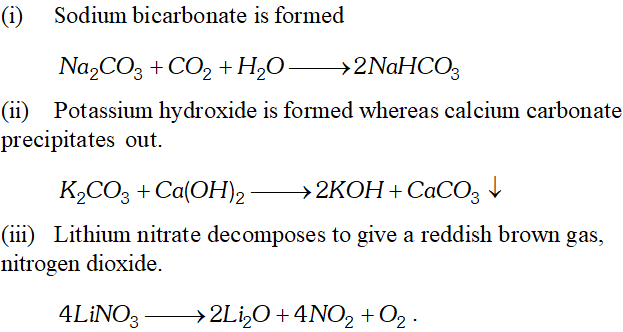Q.. What happen when (i) Magnesium is burnt in air (ii) Quick lime is heated with silica (iii) Chlorine reacts with slaked lime (iv) Calcium nitrate is heated Ans.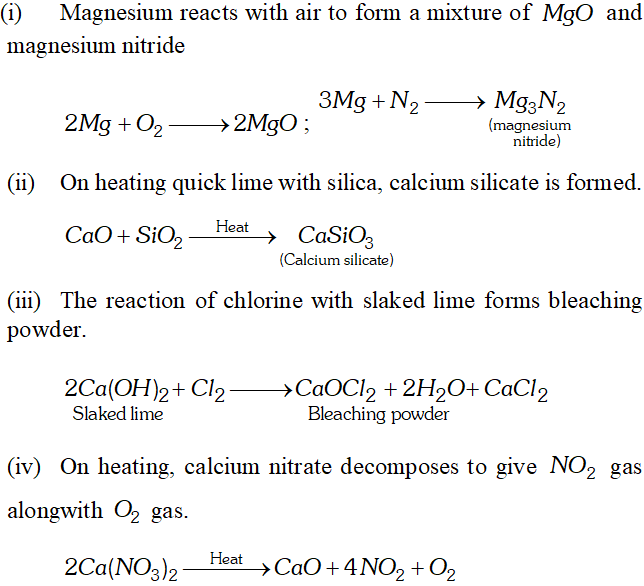Q.. ‘The chemistry of beryllium is not essentially ionic’. Justify the statement by making a reference to the nature of oxide, chloride and fluoride of beryllium. [NCERT] Ans. Be predominantly forms covalent compounds due to smaller size, higher ionisation energy and high polarising power.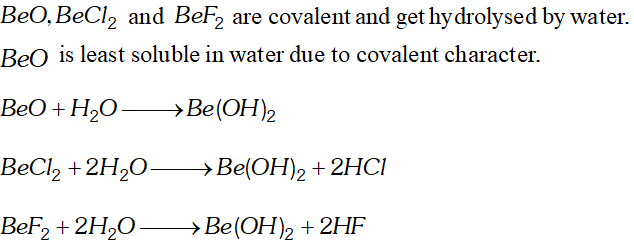$B e O, B e C l_{2}$and$B e F_{2}$are covalent and get hydrolysed by water. BeO is least soluble in water due to covalent character.$B e O+H_{2} O \longrightarrow B e(O H)_{2}B e C l_{2}+2 H_{2} O \longrightarrow B e(O H)_{2}+2 H C lB e F_{2}+2 H_{2} O \longrightarrow B e(O H)_{2}+2 H F$They are less soluble in water but more soluble in organic solvents, which shows they are covalent in nature. Q.. Compare and contrast the chemistry of group 1 metals with that of group 2 metals with respect to : (i) nature of oxides (ii) solubility and thermal stability of carbonates (iii) polarizing power of cations (iv) reactivity and reducing power. Ans. (i) Oxides of group 1 elements are more basic than that of group 2. (ii) Solubility and thermal stability of carbonates of group 1 is higher than that of group 2. (iii) Polarizing power of cations of group 2 is higher than that of group 1. (iv) Reactivity and reducing power of group 1 elements is higher than corresponding group 2 elements. Q.. Describe the importance of the following in different areas : (i) limestone (ii) cement (iii) plaster of paris. Ans. (i) Lime stone : (a) It is used in manufacture of glass and cement. (b) It is used as flux in extraction of iron. (ii) Cement : (a) It is used as building material. (b) It is used in concrete and reinforced concrete, in plastering and in construction of bridges, dams and buildings. (iii) Plaster of Paris : (a) It is used for manufacture of chalk. (b) It is used for plastering fractured bones. (c) It is used for making casts and moulds. (d) It is also used in dentistry, in ornaments work and for taking casts of statues. Q.. Describe the general characteristics of group1 elements. Ans. (i) Group-I consists of lithium, sodium, potassium, rubidium, caesium and francium. (ii) Elements have electronic configuration$n s^{1}$(iii) Elements have 1 electron in the outermost s-orbital and have a strong tendency to lose this electron, so : (a) These are highly electropositive metals. (b) Never found in free state due to their high reactivity. (c) They form$M^{+}$ion. (iv) Atomic radii : Atomic radii of alkali metals are largest in their respective periods. (v) Density : Their densities are quite low. Lithium is the lightest known metal. (vi) Oxidation state : The alkali metal atoms show only +1 oxidation state. (vii) Reducing agents : Due to very low value of ionisation energy, alkali metals are strong reducing agents and reducing character increase$N a$to$C s$but$L$but is the strongest reducing agent. (viii) When alkali metals are heated in air, Lithium forms normal oxide$\left(L i_{2} O\right)$sodium forms peroxide$\left(N a_{2} O_{2}\right)$potassium, rubidium and caesium form superoxides with peroxides. (ix) The alkali metals form hydrides of type reacting with hydrogen at about$673 K \quad \text { (Li forms hydride at } 1073 K)$forms hydride at Ionic character of hydrides increases from to Q.. Describe the manufacture process of sodium-carbonate. Ans. Sodium carbonate$\mathrm{Na}_{2} \mathrm{CO}_{3} \cdot \mathrm{H}_{2} \mathrm{O}$or washing soda is manufactured by solvay-process. Principle of process : Carbondioxide gas is passed through brine solution (about 28%$N a C l$saturated with ammonia, sodium carbonate is formed.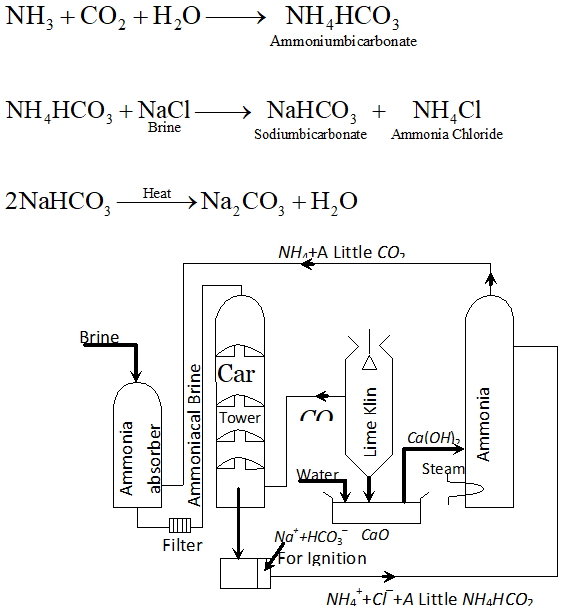Plant used for the manufacture of washing soda. Process : It completes in the following steps : (i) Saturation of brine with ammonia : Lime stone (Calcium carbonate is strongly heated to form carbon dioxide.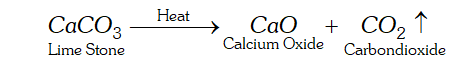Ammonia and carbondioxide mixture is passed through a tower in which saturated brine is poured down. Ammoniated brine is filtered to remove impurities of calcium and magnesium carbonate. (ii) The milky solution is removed and filtered with the help of a vacuum pump. (iii) Ammonia recovery tower : The filtrate is step 2 is mixed with calcium hydroxide and heated with steam.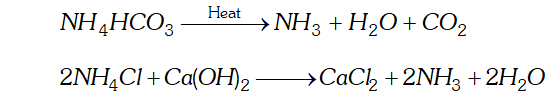Ammonia obtained is recycled with carbondioxides. Potassium carbonate cannot be prepared by Solvay process as the solubility of$\mathrm{KHCO}_{3}$is fairly large as compared to$\mathrm{NaHCO}_{3}$Q.. Give chemical equations for the various reactions taking place during the manufacture of washing soda by Solvay’s process. What are the raw materials used in this process ? What is the by-product in this process ? Ans.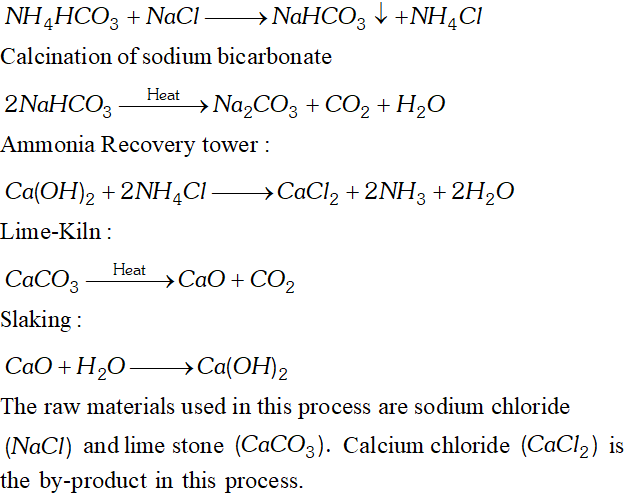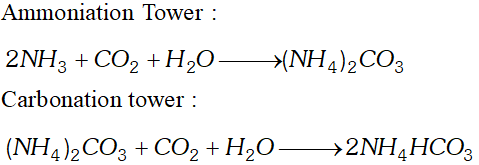The raw materials used in this process are sodium chloride and lime stone Calcium chloride is the by-product in this process. Q.. Describe three industrial uses of caustic soda. Describe one method of manufacture of sodium hydroxide. What happens when sodium hydroxides reacts with (i) aluminium metal (ii)$\mathrm{CO}_{2}$(iii)$\mathrm{SiO}_{2} ?$Ans. Industrial uses of caustic soda. (i) It is used for manufacture of soap. (ii) It is used in paper industry. (iii) It is used in textile industries. Sodium hydroxide can be prepared by electrolysis of saturted solution of brine$(N a C l)$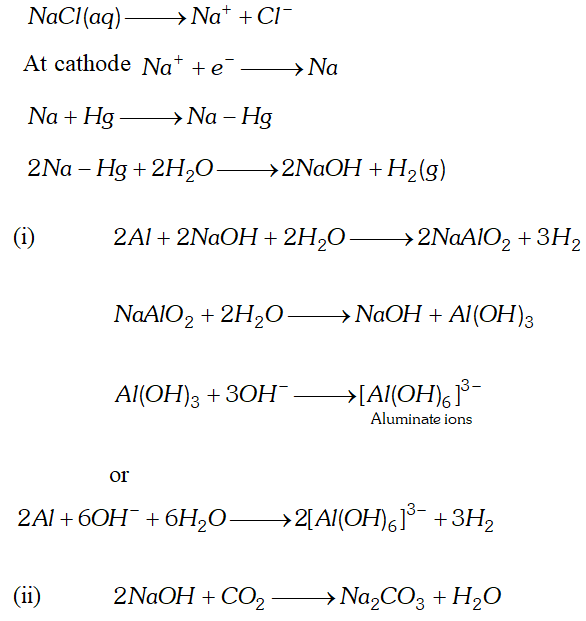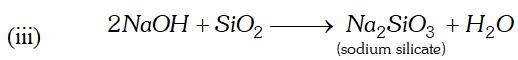(iii) Q.. (i) How is plaster of Paris prepared ? Describe its chief property due to which it is widely used. (ii) How would you explain ? (a)$\quad$BeO is insoluble but$B e S O_{4}$in soluble in water. (b)$\quad B a O$is soluble but$B a S O_{4}$is insoluble in water. (c)$\quad$Lil is more soluble than$K I$. (d)$\quad \mathrm{NaHCO}_{3}$is known in solid state but$\mathrm{Ca}\left(\mathrm{HCO}_{3}\right)_{2}$is not isolated in solid state. \ [NCERT] Ans.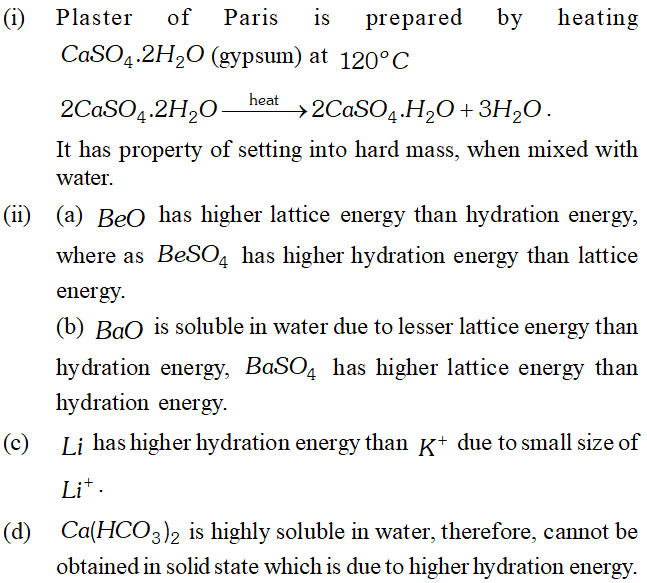Q.. (i) Name an element which is invariable bivalent and whose oxide is soluble in excess of$N a O H$and its dipositive ion has a noble gas core. (ii) Differentiate between (a) quick-lime (b) lime-water (c) slaked-lime. Ans. (i) Beryllium : It is invariably divalent. Its oxide is soluble in and its dipositive ion has a noble gas core. (ii) Quick lime : It is calcium oxide$(C a O)$It is produced by heating$\mathrm{CaCO}_{3}$Lime water : The clear aqueous solution of Calcium hydroxide in water is called lime water. It is formed when$\mathrm{Ca}(\mathrm{OH})_{2}$is dissolved in excess of$H_{2} O$Slaked Lime : Calcium hydroxide solid is known as Slaked Lime. It is produced when water is added to$\mathrm{CaO}$Q.. What is the effect of heat on the following compounds ? (Write equations for the reactions). (i) Calcium carbonate. (ii) Magnesium chloride hexahydrate. (iii) Gypsum. (iv) Magnesium sulphate heptahydrate. Ans. (i) Action of heat on calcium carbonate : When calcium carbonate is heated, a colourless gas carbon dioxide is given out and a white residue of calcium oxide is left behind.(ii) Action of heat on magnesium chloride hexahydrate : It loses water and hydrogen chloride and yields a residue of magnesium oxide.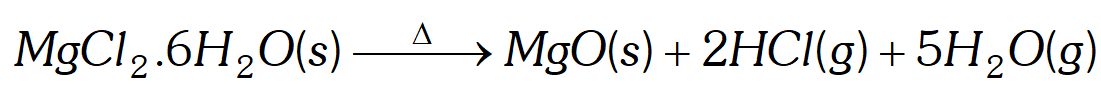(iii) Action of heat on gypsum : It forms a hemi-hydrate, called plaster of paris.(iv) Action of heat on magnesium sulphate heptahydrate : It loses water of crystallization and forms anhydrous salt.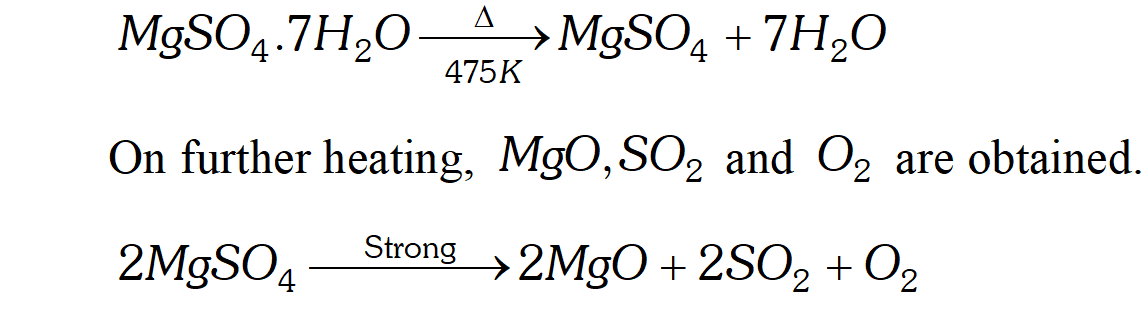Q.. (i) Describe two important uses of each of the following: (a) caustic soda (b) sodium carbonate (c) quicklime.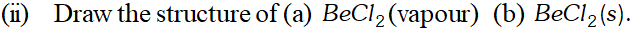Ans. (i) (a) Caustic soda is used in prepartion of pure fats and oils. It is also used for preparation of rayon (artificial silk). (b) Sodium carbonate is used for manufacture of glass. It is used in softening of hard water. (c) Quick lime is used for white washing. It is used for manufacturing of glass and cement.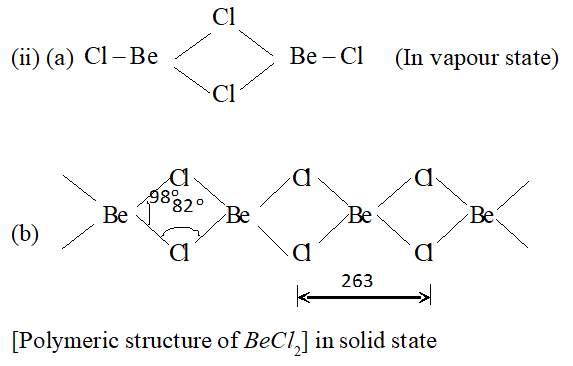[Polymeric structure of$\left.B e C l_{2}\right]\$ in solid state

Student
March 9, 2022, 9:11 p.m.
Nice
Faltu
March 9, 2022, 9:09 p.m.
Nice
Nav
Feb. 24, 2022, 1:06 p.m.
Awesome!
Babli
May 13, 2021, 10:27 a.m.
Vvvvv nice 👌👌👌👌👌
oilless compressor
April 13, 2021, 10:29 a.m.
I loved your blog and thanks for publishing this about s block elements class 11 important questions with answers! I am really happy to come across this exceptionally well written content. Thanks for sharing and look for more in future!! Keep doing this inspirational work and share with us. I have also found this resource https://peerlessengineering.com/ useful and its related to what you are mentioning.
Student
March 10, 2021, 12:07 a.m.
Thank you, helped in last minute preparation.
Priyanka
Jan. 16, 2021, 7:46 p.m.
It is very good questions